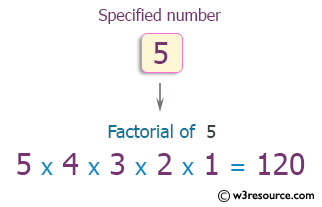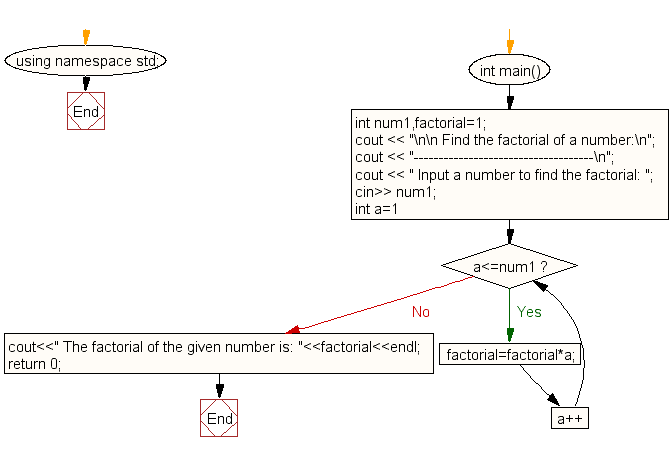﻿ C++ Exercises: Find the factorial of a number - w3resource# C++ Exercises: Find the factorial of a number

## C++ For Loop: Exercise-7 with Solution

Write a program in C++ to find the factorial of a number.

Pictorial Presentation:Sample Solution :-

C++ Code :

``````#include <iostream>
using namespace std;
int main()
{
int num1,factorial=1;
cout << "\n\n Find the factorial of a number:\n";
cout << "------------------------------------\n";
cout << " Input a number to find the factorial: ";
cin>> num1;
for(int a=1;a<=num1;a++)
{
factorial=factorial*a;
}
cout<<" The factorial of the given number is: "<<factorial<<endl;
return 0;
}
``````

Sample Output:

``` Find the factorial of a number:
------------------------------------
Input a number to find the factorial: 5
The factorial of the given number is: 120
```

Flowchart:C++ Code Editor:

Contribute your code and comments through Disqus.

What is the difficulty level of this exercise?

﻿